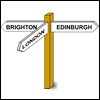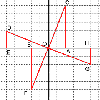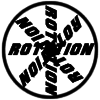# Resources tagged with: Matrices

Filter by: Content type:
Age range:
Challenge level:

There are 13 NRICH Mathematical resources connected to Matrices, you may find related items under Vectors and Matrices.

Broad Topics > Vectors and Matrices > Matrices### Limiting Probabilities

##### Age 16 to 18Challenge Level

Given probabilities of taking paths in a graph from each node, use matrix multiplication to find the probability of going from one vertex to another in 2 stages, or 3, or 4 or even 100.### 8 Methods for Three by One

##### Age 14 to 18Challenge Level

This problem in geometry has been solved in no less than EIGHT ways by a pair of students. How would you solve it? How many of their solutions can you follow? How are they the same or different?. . . .### Nine Eigen

##### Age 16 to 18Challenge Level

Explore how matrices can fix vectors and vector directions.### Matrix Meaning

##### Age 16 to 18Challenge Level

Explore the meaning behind the algebra and geometry of matrices with these 10 individual problems.### Square Pair

##### Age 16 to 18Challenge Level

Explore the shape of a square after it is transformed by the action of a matrix.### Transformations for 10

##### Age 16 to 18Challenge Level

Explore the properties of matrix transformations with these 10 stimulating questions.### Fix Me or Crush Me

##### Age 16 to 18Challenge Level

Can you make matrices which will fix one lucky vector and crush another to zero?### Two and Four Dimensional Numbers

##### Age 16 to 18Challenge Level

Investigate matrix models for complex numbers and quaternions.### The Golden Ratio, Fibonacci Numbers and Continued Fractions.

##### Age 14 to 16

An iterative method for finding the value of the Golden Ratio with explanations of how this involves the ratios of Fibonacci numbers and continued fractions.### The Matrix

##### Age 16 to 18Challenge Level

Investigate the transfomations of the plane given by the 2 by 2 matrices with entries taking all combinations of values 0. -1 and +1.### Stringing it Out

##### Age 14 to 16Challenge Level

Explore the transformations and comment on what you find.### Reflect Again

##### Age 16 to 18Challenge Level

Follow hints to investigate the matrix which gives a reflection of the plane in the line y=tanx. Show that the combination of two reflections in intersecting lines is a rotation.### Rots and Refs

##### Age 16 to 18Challenge Level

Follow hints using a little coordinate geometry, plane geometry and trig to see how matrices are used to work on transformations of the plane.